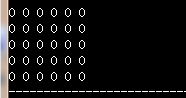# 【c++学习笔记四】浅析vector容器的实例

## 二、基本函数实现

### 1.构造函数

• vector():创建一个空vector
• vector(int nSize):创建一个vector,元素个数为nSize
• vector(int nSize,const t& t):创建一个vector，元素个数为nSize,且值均为t
• vector(const vector&):复制构造函数
• vector(begin,end):复制[begin,end)区间内另一个数组的元素到vector中

### 2.增加函数

• void push_back(const T& x):向量尾部增加一个元素X
• iterator insert(iterator it,const T& x):向量中迭代器指向元素前增加一个元素x
• iterator insert(iterator it,int n,const T& x):向量中迭代器指向元素前增加n个相同的元素x
• iterator insert(iterator it,const_iterator first,const_iterator last):向量中迭代器指向元素前插入另一个相同类型向量的[first,last)间的数据

### 3.删除函数

• iterator erase(iterator it):删除向量中迭代器指向元素
• iterator erase(iterator first,iterator last):删除向量中[first,last)中元素
• void pop_back():删除向量中最后一个元素
• void clear():清空向量中所有元素

### 4.遍历函数

• reference at(int pos):返回pos位置元素的引用
• reference front():返回首元素的引用
• reference back():返回尾元素的引用
• iterator begin():返回向量头指针，指向第一个元素
• iterator end():返回向量尾指针，指向向量最后一个元素的下一个位置
• reverse_iterator rbegin():反向迭代器，指向最后一个元素
• reverse_iterator rend():反向迭代器，指向第一个元素之前的位置

### 5.判断函数

• bool empty() const:判断向量是否为空，若为空，则向量中无元素

### 6.大小函数

• int size() const:返回向量中元素的个数
• int capacity() const:返回当前向量张红所能容纳的最大元素值
• int max_size() const:返回最大可允许的vector元素数量值

### 7.其他函数

• void swap(vector&):交换两个同类型向量的数据
• void assign(int n,const T& x):设置向量中第n个元素的值为x
• void assign(const_iterator first,const_iterator last):向量中[first,last)中元素设置成当前向量元素

### 8.看着清楚

1.push_back 在数组的最后添加一个数据
2.pop_back 去掉数组的最后一个数据
3.at 得到编号位置的数据
4.begin 得到数组头的指针
5.end 得到数组的最后一个单元+1的指针
6．front 得到数组头的引用
7.back 得到数组的最后一个单元的引用
8.max_size 得到vector最大可以是多大
9.capacity 当前vector分配的大小
10.size 当前使用数据的大小
11.resize 改变当前使用数据的大小，如果它比当前使用的大，者填充默认值
12.reserve 改变当前vecotr所分配空间的大小
13.erase 删除指针指向的数据项
14.clear 清空当前的vector
15.rbegin 将vector反转后的开始指针返回(其实就是原来的end-1)
16.rend 将vector反转构的结束指针返回(其实就是原来的begin-1)
17.empty 判断vector是否为空
18.swap 与另一个vector交换数据

## 三、基本用法

#include < vector>
using namespace std;

## 四、简单介绍

1. Vector<类型>标识符
2. Vector<类型>标识符(最大容量)
3. Vector<类型>标识符(最大容量,初始所有值)
4. Int i={1,2,3,4,5}
Vector<类型>vi(I,i+2);//得到i索引值为3以后的值
5. Vector< vector< int> >v; 二维向量//这里最外的<>要有空格。否则在比较旧的编译器下无法通过

## 实例来咯

### 1.pop_back()&push_back(elem)实例在容器最后移除和插入数据

``````#include <string.h>
#include <vector>
#include <iostream>
using namespace std;

int main()
{
vector<int>obj(10,0);//最大容器为10，都初始化为0
for(int i=0;i<10;i++)//push_back(elem)在数组最后添加数据
{
obj.push_back(i);
cout<<obj[i]<<",";
}

for(int i=0;i<5;i++)//去掉数组最后一个数据
{
obj.pop_back();
}

cout<<"\n"<<endl;

for(int i=0;i<obj.size();i++)//size()容器中实际数据个数
{
cout<<obj[i]<<",";
}

return 0;
}``````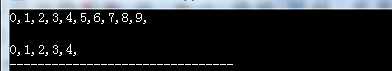### 2.clear()清除容器中所以数据

``````#include <string.h>
#include <vector>
#include <iostream>
using namespace std;

int main()
{
vector<int>obj;
for(int i=0;i<10;i++)//push_back(elem)在数组最后添加数据
{
obj.push_back(i);
cout<<obj[i]<<",";
}

obj.clear();//清除容器中所以数据
for(int i=0;i<obj.size();i++)
{
cout<<obj[i]<<endl;
}

return 0;
}``````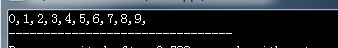### 3.排序

``````#include <string.h>
#include <vector>
#include <iostream>
#include <algorithm>
using namespace std;

int main()
{
vector<int>obj;

obj.push_back(1);
obj.push_back(3);
obj.push_back(0);

sort(obj.begin(),obj.end());//从小到大

cout<<"从小到大:"<<endl;
for(int i=0;i<obj.size();i++)
{
cout<<obj[i]<<",";
}

cout<<"\n"<<endl;

cout<<"从大到小:"<<endl;
reverse(obj.begin(),obj.end());//从大到小
for(int i=0;i<obj.size();i++)
{
cout<<obj[i]<<",";
}
return 0;
}
``````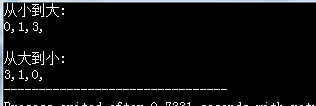1.注意sort需要头文件#include < algorithm>
2.如果想sort来降序，可重写sort
bool compare(int a,int b)
{
return a< b; //升序排列，如果改为return a>b，则为降序
}
int a={2,4,1,23,5,76,0,43,24,65},i;
for(i=0;i<20;i++)
cout<< a[i]<< endl;
sort(a,a+20,compare);

### 4.访问（直接数组访问&迭代器访问）

``````#include <string.h>
#include <vector>
#include <iostream>
#include <algorithm>
using namespace std;

int main()
{
//顺序访问
vector<int>obj;
for(int i=0;i<10;i++)
{
obj.push_back(i);
}

cout<<"直接利用数组：";
for(int i=0;i<10;i++)//方法一
{
cout<<obj[i]<<" ";
}

cout<<endl;
cout<<"利用迭代器：" ;
//方法二，使用迭代器将容器中数据输出
vector<int>::iterator it;//声明一个迭代器，来访问vector容器，作用：遍历或者指向vector容器的元素
for(it=obj.begin();it!=obj.end();it++)
{
cout<<*it<<" ";
}
return 0;
}``````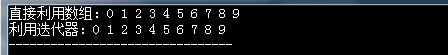### 5.二维数组两种定义方法（结果一样）

``````#include <string.h>
#include <vector>
#include <iostream>
#include <algorithm>
using namespace std;

int main()
{
int N=5, M=6;
vector<vector<int> > obj(N); //定义二维动态数组大小5行
for(int i =0; i< obj.size(); i++)//动态二维数组为5行6列，值全为0
{
obj[i].resize(M);
}

for(int i=0; i< obj.size(); i++)//输出二维动态数组
{
for(int j=0;j<obj[i].size();j++)
{
cout<<obj[i][j]<<" ";
}
cout<<"\n";
}
return 0;
}``````

``````#include <string.h>
#include <vector>
#include <iostream>
#include <algorithm>
using namespace std;

int main()
{
int N=5, M=6;
vector<vector<int> > obj(N, vector<int>(M)); //定义二维动态数组5行6列

for(int i=0; i< obj.size(); i++)//输出二维动态数组
{
for(int j=0;j<obj[i].size();j++)
{
cout<<obj[i][j]<<" ";
}
cout<<"\n";
}
return 0;
}``````